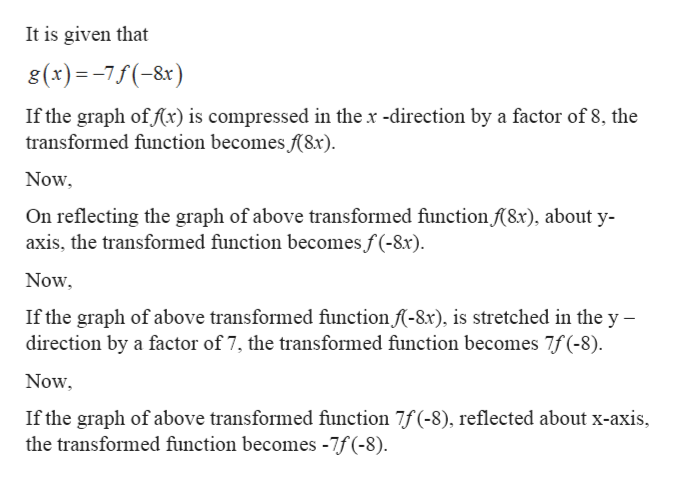# Consider the transformation of the function f(x)f(x) given byg(x)=−7f(−8x).g(x)=−7f(−8x).If the point (−10,4)(−10,4) is on the graph of f(x)f(x), what point must be on the graph of g(x)g(x)?

Question
27 views

Consider the transformation of the function f(x)f(x) given by

g(x)=−7f(−8x).g(x)=−7f(−8x).

If the point (−10,4)(−10,4) is on the graph of f(x)f(x), what point must be on the graph of g(x)g(x)?

check_circle

Step 1

Given: -

Step 2

To find: -

Step 3

Calculation...help_outlineImage TranscriptioncloseIt is given that 8(x)=-7f(-8x) If the graph of (x) is compressed in the x -direction by a factor of 8, the transformed function becomes f(8x). Now, On reflecting the graph of above transformed function (8x), about y- axis, the transformed function becomes f(-8x). Now, If the graph of above transformed function f-8x), is stretched in the y – direction by a factor of 7, the transformed function becomes 7f (-8). Now, If the graph of above transformed function 7f (-8), reflected about x-axis, the transformed function becomes -7f (-8). fullscreen

### Want to see the full answer?

See Solution

#### Want to see this answer and more?

Solutions are written by subject experts who are available 24/7. Questions are typically answered within 1 hour.*

See Solution
*Response times may vary by subject and question.
Tagged in

### Other